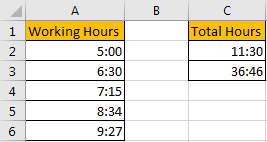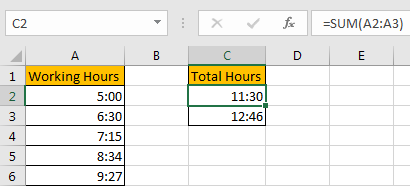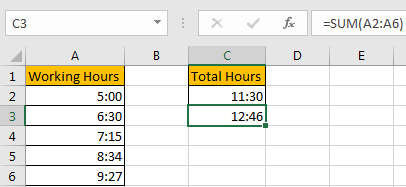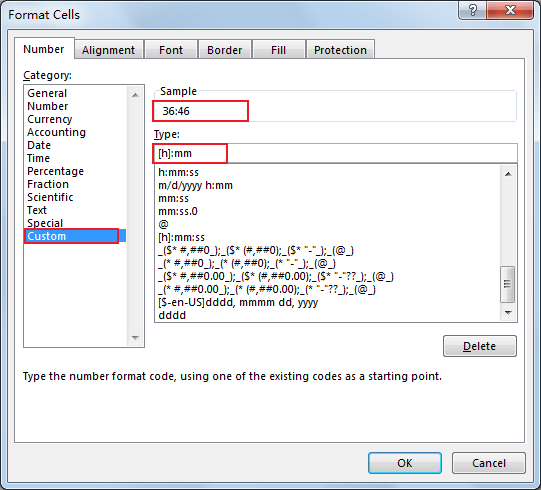# How to Add Times Over 24 Hours in Excel

When calculating the total working hours or total efforts in our work, we can use SUM function to calculate total hours conveniently. But when you applying this function in you daily work, you may notice that it only works to calculate total hours less than 24 hours, if the total hours are greater than 24 hours, it cannot work normally and we may get an improper returned value.

See example below.

1.Total hours is calculated correctly when applying SUM for A2 and A3.2.Total hours is calculated incorrectly when applying SUM for A2 to A6.## Method: Add Times Over 24 Hours by Format Cells Feature

Step 1: Enter the formula =SUM(A2:A6) in B3 to get total hours for provided working hours. Refer to above screenshot we can get the returned value 12:46, obviously it is incorrect.

Step 2: Select this cell, right click to load options, select Format Cells in the last section.Step 3: On Format Cells panel, under Number tab, select Custom in Category list, in Type textbox type ‘[h]:mm’, after typing you can see in Sample section ’36:46’ is displayed. If your time format is hh:mm:ss in working hours, you can enter ‘[h]:mm:ss’ in Type.Step 4: Click OK. Verify that total hours for A2:A6 is updated properly.### Related Functions

• Excel SUM function
The Excel SUM function will adds all numbers in a range of cells and returns the sum of these values. You can add individual values, cell references or ranges in excel.The syntax of the SUM function is as below:= SUM(number1,[number2],…)…